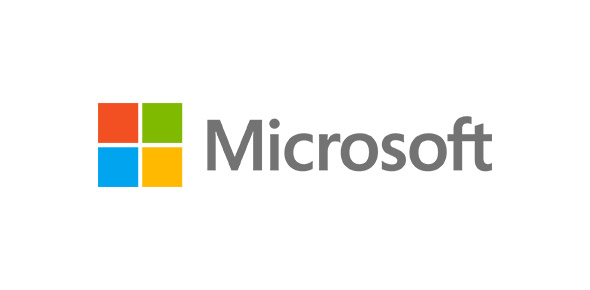# Microsoft Excel Proficiency Trivia Questions

30 Questions | Total Attempts: 61SettingsThis quiz is created to identify the proficiency of users about Microsoft Excel.

• 1.
The number of rows in an Excel 2007 worksheet is:
• A.

65536

• B.

256

• C.

64536

• D.

1048576

• 2.
Reference to the above image of Command Group "Alignment" which of the following tabs consist it:
• A.

View

• B.

Formatting

• C.

Home

• 3.
You can have results of any database table into Excel Worksheet, and by one click you can refresh the results?
• A.

True

• B.

False

• 4.
When using a databar, changing the value of one cell in the range will not affect the barsize of all the other cells.
• A.

True

• B.

False

• 5.
The Insert Function dialog box tells you how to use functions.
• A.

True

• B.

False

• 6.
When a worksheet is printed, the grid lines that surround the cells are printable by default.
• A.

True

• B.

False

• 7.
When you clear a filter, all filters in the worksheet are cleared, if worksheet contains more than one filters
• A.

True

• B.

False

• 8.
The function arguments dialog box tells you how to use functions.
• A.

True

• B.

False

• 9.
The simplest and most convenient way to add a record to a table is to enter the data in the first blank row.
• A.

True

• B.

False

• 10.
If you insert a new row at the top of the table, the new row becomes the header row and is formatted with the table style.
• A.

True

• B.

False

• 11.
In Excel, charts do not remain linked or connected to the data sources if they appear in different worksheets.
• A.

True

• B.

False

• 12.
After you merge a range into a single cell, you can't realign its content.
• A.

True

• B.

False

• 13.
Once you hide a row, it remains hidden as long as the workbook is active.
• A.

True

• B.

False

• 14.
All functions have arguments.  There are no functions which work without providing them arguments.
• A.

True

• B.

False

• 15.
Although 3D Charts are visually attractive, they can obscure the relationship between the values in the chart by making it difficult to see when slice is larger.
• A.

True

• B.

False

• 16.
Excel has a builtin format to display 4 digit year values, so you do not need to create one.
• A.

True

• B.

False

• 17.
Formatting data only change the appearance of data, it does not affect the data itself.
• A.

True

• B.

False

• 18.
If a formula contains several functions, Excel starts with the outermost function and then moves inward.
• A.

True

• B.

False

• 19.
An easy way to sort data when there is only one sort field is to use the Sort A to Z or Sort Z to A buttons.
• A.

True

• B.

False

• 20.
You can create maximum one Excel table in a worksheet
• A.

True

• B.

False

• 21.
As you begin to type a function name within a formula into a cell, a list of functions that begin with the letters you typed appears.
• A.

True

• B.

False

• 22.
You can rotate a 3D chart in only two directions: horizontally along the x-axis and vertically along the y-axis.
• A.

True

• B.

False

• 23.
Pressing ENTER moves the selection one cell to the right by default.
• A.

True

• B.

False

• 24.
Ctrl+B key makes the shortcut for making font in selected cell bold.
• A.

True

• B.

False

• 25.
What key should be used to edit the content of a cell?
• A.

F1

• B.

F2

• C.

F3

Related TopicsBack to top